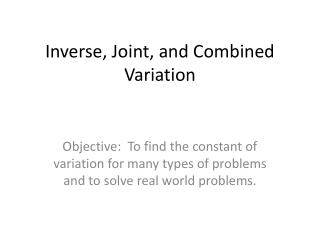DownloadDownload PresentationInverse, Joint, and Combined Variation

# Inverse, Joint, and Combined Variation

Télécharger la présentation## Inverse, Joint, and Combined Variation

- - - - - - - - - - - - - - - - - - - - - - - - - - - E N D - - - - - - - - - - - - - - - - - - - - - - - - - - -
##### Presentation Transcript

1. Inverse, Joint, and Combined Variation Objective: To find the constant of variation for many types of problems and to solve real world problems.

2. Inverse Variation • Two variables, x and y, have an inverse-variation relationship if there is a nonzero number k such that xy = k, y = k/x. The constant of variation is k.

3. Example 1

4. Example 1

5. Example 1

6. Try This • The variable y varies inversely as x, and y = 120 when x = 6.5. Find the constant of variation and write an equation for the relationship. Then, find y when x is 1.5, 4.5, 8, 12.5, and 14.

7. Try This • The variable y varies inversely as x, and y = 120 when x = 6.5. Find the constant of variation and write an equation for the relationship. Then, find y when x is 1.5, 4.5, 8, 12.5, and 14.

8. Try This • The variable y varies inversely as x, and y = 120 when x = 6.5. Find the constant of variation and write an equation for the relationship. Then, find y when x is 1.5, 4.5, 8, 12.5, and 14.

9. Joint Variation • If y = kxz, then y varies jointly as x and z, and the constant of variation is k.

10. Example 2

11. Example 2

12. Squared Variation • If , where k is a nonzero constant, then y varies directly as the square of x. Many geometric relationships involve this type of variation, as show in the next example.

13. Example 3

14. Example 3

15. Example 3

16. Try This • Write the formula for the area A, of a circle whose radius is r. Identify the type of variation and the constant of variation. • Find the area of the circle when r is 1.5, 2.5, 3.5, 4.5.

17. Try This • Write the formula for the area A, of a circle whose radius is r. Identify the type of variation and the constant of variation. • Find the area of the circle when r is 1.5, 2.5, 3.5, 4.5. • The constant of variation is .

18. Try This • Write the formula for the area A, of a circle whose radius is r. Identify the type of variation and the constant of variation. • Find the area of the circle when r is 1.5, 2.5, 3.5, 4.5. • The constant of variation is .

19. Combined Variation

20. Example 4

21. Example 4

22. Example 4

23. Homework • Page 486 • 13-27 odd